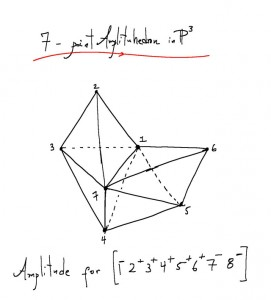# Amplituhedron

Source: Quanta, Sep 2013

… a newly discovered mathematical object resembling a multifaceted jewel in higher dimensions. Encoded in its volume are the most basic features of reality that can be calculated — the probabilities of outcomes of particle interactions.

…  dramatically simplifies calculations of particle interactions and challenges the notion that space and time are fundamental components of reality.The revelation that particle interactions, the most basic events in nature, may be consequences of geometry significantly advances a decades-long effort to reformulate quantum field theory, the body of laws describing elementary particles and their interactions. Interactions that were previously calculated with mathematical formulas thousands of terms long can now be described by computing the volume of the corresponding jewel-like “amplituhedron,” which yields an equivalent one-term expression.

“The degree of efficiency is mind-boggling,” said Jacob Bourjaily, a theoretical physicist at Harvard University and one of the researchers who developed the new idea. “You can easily do, on paper, computations that were infeasible even with a computer before.”The 60-year-old method for calculating scattering amplitudes — a major innovation at the time — was pioneered by the Nobel Prize-winning physicist Richard Feynman. He sketched line drawings of all the ways a scattering process could occur and then summed the likelihoods of the different drawings.

“The number of Feynman diagrams is so explosively large that even computations of really simple processes weren’t done until the age of computers,” Bourjaily said.

A seemingly simple event, such as two subatomic particles called gluons colliding to produce four less energetic gluons (which happens billions of times a second during collisions at the Large Hadron Collider), involves 220 diagrams, which collectively contribute thousands of terms to the calculation of the scattering amplitude.

In 1986, it became apparent that Feynman’s apparatus was a Rube Goldberg machine.

To prepare for the construction of the Superconducting Super Collider in Texas (a project that was later canceled), theorists wanted to calculate the scattering amplitudes of known particle interactions to establish a background against which interesting or exotic signals would stand out. But even 2-gluon to 4-gluon processes were so complex, a group of physicists had written two years earlier, “that they may not be evaluated in the foreseeable future.”

… based on a pattern they observed in the scattering amplitudes of other gluon interactions, Parke and Taylor guessed a simple one-term expression for the amplitude. It was, the computer verified, equivalent to the 9-page formula. In other words, the traditional machinery of quantum field theory, involving hundreds of Feynman diagrams worth thousands of mathematical terms, was obfuscating something much simpler. As Bourjaily put it: “Why are you summing up millions of things when the answer is just one function?”

In the mid-2000s, more patterns emerged in the scattering amplitudes of particle interactions, repeatedly hinting at an underlying, coherent mathematical structure behind quantum field theory.A sketch of the amplituhedron representing an 8-gluon particle interaction. Using Feynman diagrams, the same calculation would take roughly 500 pages of algebra.

Beyond making calculations easier or possibly leading the way to quantum gravity, the discovery of the amplituhedron could cause an even more profound shift, Arkani-Hamed said. That is, giving up space and time as fundamental constituents of nature and figuring out how the Big Bang and cosmological evolution of the universe arose out of pure geometry.

This site uses Akismet to reduce spam. Learn how your comment data is processed.